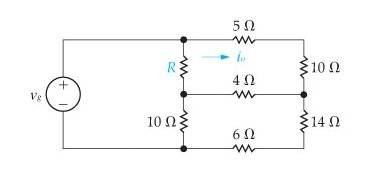# Circuit Analysis

• Engineering
Gold Member

## Homework StatementThe current i_o = 8A, Voltage v_g = 480 V.

a) Find the value of R.

b) Find the power supplied by the voltage source.

## The Attempt at a Solution

Well, I noted both pairs of series resistors, to make the calculations a little simpler, and what I ended up with was this delta-Y or Y-delta formation (not sure, just saw something about it on a post here while digging around).

I figured that the voltage drops across each resistor MUST equal 480V, so I started setting up the equations, but I'm not sure if I really did it right.

There were 5 other currents which are not given. I dubbed the one passing through 'R' to be I3, the one passing through the 4ohm resistor to be I1, the one passing through the 20ohm resistor series as I2, and the one passing through the 10ohm resistor as I4. I dubbed the current flowing through the voltage source to be Is.

Doing what I hoped was an accurate KVL of that freakin triangle thing, as well as some KCLs, I came up with 5 equations, with a total of 6 variables including the Resistor....
At this point I'm pretty sure I've done something wrong, but I'm not sure what?

Part B should be easy once I find Part A.

Gold Member
2021 Award
I figured that the voltage drops across each resistor MUST equal 480V

Huh ??? You need to be a lot more specific. The exact statement you made does not make sense.

Gold Member
Sorry, I meant the voltage drop across the entire resistor mass, that whole freaky mess of resistors that I don't understand. Not each resistor.

Gold Member
2021 Award
Sorry, I meant the voltage drop across the entire resistor mass, that whole freaky mess of resistors that I don't understand. Not each resistor.

OK, that makes sense. Now you need to lable your figure to go with your work. When you are asking someone to help you out, it's not a great idea to make it hard for them.

Also, I'd suggest just doing it by loops. Forget about deltas and Ys

Mentor
There's enough information given to work your way through the circuit, placing node voltages and currents as you go. For example, you're given that ##i_o = 8A##. So what must be the voltage drop across the 15Ω it runs through? So what then is the following node's potential?(assume Vg's negative terminal is a common reference point)

Gold Member
OK, that makes sense. Now you need to lable your figure to go with your work. When you are asking someone to help you out, it's not a great idea to make it hard for them.

Also, I'd suggest just doing it by loops. Forget about deltas and Ys

Actually, The figure is labeled. Right below the picture I put in the values.

There's enough information given to work your way through the circuit, placing node voltages and currents as you go. For example, you're given that ##i_o = 8A##. So what must be the voltage drop across the 15Ω it runs through? So what then is the following node's potential?(assume Vg's negative terminal is a common reference point)

It's a voltage drop of 120V altogether. But from there on it turns into a huge mess of equations :(

But we just learned the Delta-Y formulas in class yesterday, so hopefully that will make this easier.

EDIT: Using the delta-y method made the system much easier to solve. yielded 3 equations with 3 variables, as opposed to the 6 or 7 I had before!

Last edited:
Mentor
Actually, The figure is labeled. Right below the picture I put in the values.

It's a voltage drop of 120V altogether. But from there on it turns into a huge mess of equations :(

But we just learned the Delta-Y formulas in class yesterday, so hopefully that will make this easier.

EDIT: Using the delta-y method made the system much easier to solve. yielded 3 equations with 3 variables, as opposed to the 6 or 7 I had before!

You didn't need to deal with "a huge mess of equations". With the voltage drop of 120V you have the potential at the next node, and thus the current through the 14Ω + 6Ω resistors. A little KCL at the node and you've got the current through the 4Ω resistor, and thus the potential at its other end, then the rest of the currents fall into place and the value of R becomes trivial.